Thank you for visiting nature.com. You are using a browser version with limited support for CSS. To obtain the best experience, we recommend you use a more up to date browser (or turn off compatibility mode in Internet Explorer). In the meantime, to ensure continued support, we are displaying the site without styles and JavaScript.

# Hydrodynamic phonon transport in suspended graphene

## Abstract

Recent studies of thermal transport in nanomaterials have demonstrated the breakdown of Fourier’s law through observations of ballistic transport. Despite its unique features, another instance of the breakdown of Fourier’s law, hydrodynamic phonon transport, has drawn less attention because it has been observed only at extremely low temperatures and narrow temperature ranges in bulk materials. Here, we predict on the basis of first-principles calculations that the hydrodynamic phonon transport can occur in suspended graphene at significantly higher temperatures and wider temperature ranges than in bulk materials. The hydrodynamic transport is demonstrated through drift motion of phonons, phonon Poiseuille flow and second sound. The significant hydrodynamic phonon transport in graphene is associated with graphene’s two-dimensional features. This work opens a new avenue for understanding and manipulating heat flow in two-dimensional materials.

## Introduction

The transport of phonons, which are the dominant heat carriers in non-metallic solids, is usually diffusive and describable by Fourier’s law of heat conduction. Regimes where Fourier’s law breaks down, such as ballistic1 and hydrodynamic2 phonon transport, were discovered in bulk materials more than 50 years ago, but these phenomena were observed only at extremely low temperatures3,4,5. Recent studies of low-dimensional materials, however, have highlighted the practical importance of ballistic phonon transport in applications such as thermoelectric materials6 and electronic devices7,8. In this work, we discuss how hydrodynamic phonon transport as well as ballistic phonon transport can be significant in a two-dimensional material, particularly in graphene.

The term hydrodynamic phonon transport arose from its similarity with macroscopic transport phenomena in fluids. In fluid flow, mass transport is mainly due to the macroscopic motion of molecules with a drift velocity. Likewise, phonons in the hydrodynamic regime exhibit macroscopic drift motion. In this sense, hydrodynamic phonon transport is different from the more well-known diffusive or ballistic phonon transport. During diffusive transport, heat is transferred through multiple scattering events among phonons without macroscopic drift motion. During ballistic transport, it is assumed that there is no internal scattering. Hydrodynamic transport, on the other hand, includes many phonon scattering events. The drift motion of phonons in the hydrodynamic regime causes two interesting hydrodynamic transport phenomena that cannot occur in either diffusive or ballistic regimes: phonon Poiseuille flow (Fig. 1a) and second sound (Fig. 1c), which are analogous to Poiseuille flow and ordinary sound in a fluid, respectively, which will be discussed later.Figure 1: The different macroscopic transport phenomena in hydrodynamic and diffusive regimes.

Despite the interesting features of hydrodynamic phonon transport, the temperature range in which it was observed was too low and narrow to consider for practical applications. For example, the reported temperature range for phonon Poiseuille flow is 0.5–1.0 K (ref. 5) and for second sound the range is 10–20 K (ref. 9). The extremely stringent temperature conditions for hydrodynamic phonon transport are due to Umklapp scattering (hereafter U-scattering), which destroys the crystal momentum. In contrast, scatterings between molecules conserve total momentum. As such, for hydrodynamic transport to occur, U-scattering should be negligibly weak compared with the other three-phonon scattering, which conserves crystal momentum, normal scattering (hereafter N-scattering). One way to suppress U-scattering is to consider low temperatures, but at too low temperatures transport becomes ballistic without internal scattering, leaving only a very narrow temperature range for hydrodynamic transport. In addition to U-scattering, the scattering of phonons by impurities such as isotopes does not conserve crystal momentum, and isotope enrichment imposes another difficulty for hydrodynamic phonon transport (hereafter R-scattering denotes Umklapp and isotope scatterings).

Here, we show that suspended graphene (hereafter graphene), unlike three-dimensional materials, is remarkably well suited for hydrodynamic phonon transport. Using first-principles calculations, we show drift motion of phonons, phonon Poiseuille flow and second sound in graphene at significantly higher and wider temperature ranges compared with those seen in three-dimensional materials. Then, we discuss how the significant hydrodynamic phonon transport in graphene stems from its two-dimensional features.

## Results

### Displaced distribution function

An indication of the hydrodynamic transport, the macroscopic drift motion, can be found in the distribution function. For example, for molecules in fluid flow, the Maxwell distribution displaced by a drift velocity is considered an equilibrium distribution10. Similarly, if there is no R-scattering among phonons, the displaced Bose–Einstein distribution has been commonly assumed for the equilibrium distribution of phonons11.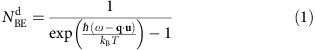where ħ, ω and q represent the Planck constant, phonon frequency and phonon wavevector, respectively. The drift velocity is represented by the displacement, u, in the phonon distribution. In the displaced distribution, the displacement is a constant for all phonon modes regardless of polarization and wavevector, describing the macroscopic drift motion of phonons having the same velocity. Assuming a small drift velocity, this displaced distribution can be linearized to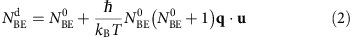where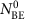is the Bose–Einstein distribution.

On the basis of the assumption of the displaced phonon distribution in the absence of R-scattering, past work derived macroscopic governing equations that describe hydrodynamic phonon transport12. However, it has remained elusive to our knowledge whether the absence of R-scattering necessarily leads to the displaced distribution. Moreover, the validity of the displaced distribution in real materials, where R-scattering cannot be completely avoided, has not been explicitly confirmed. In this work, we demonstrate from first-principles calculations that a displaced distribution of phonons can occur in graphene even in the presence of weak R-scattering, which validates the assumption of the displaced distribution used in the past studies12,13 and correspondingly shows hydrodynamic phonon transport in graphene. The recent progress in calculating the phonon–phonon scattering matrix using first-principles14,15 and numerical methods for solving the Peierls–Boltzmann equation16,17 enable the calculation of phonon distributions in a specific material under a temperature gradient. This first principles-based method has been demonstrated to be highly accurate and have predictive power for phonon transport in many materials including graphene15,18,19,20,21,22,23. In this work, to capture the significant role of N-scattering, we solve the full solution of the Peierls–Boltzmann equation without relaxation time approximation.

In Fig. 2, we show this phonon distribution in an infinitely large graphene sheet with 0.1% concentration of isotope 13C at 100 K under a static temperature gradient. The temperature gradient is required to maintain the drift motion since R-scattering cannot be completely ignored in real materials. Figure 2 plots the normalized deviation of the distribution from the stationary equilibrium distribution, defined as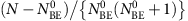, to examine the existence of a drift velocity. It is clearly seen in Fig. 2b that the normalized deviation is linear to wavevector qx along the temperature gradient direction with the same slope for all three acoustic branches in a wide range of wavevectors. This indicates that the distribution is indeed displaced, giving the macroscopic motion of phonons with the same velocity as in equation (2). In usual cases without strong hydrodynamic transport features, the normalized deviation shows no correlation among phonon modes and phonons cannot exhibit any macroscopic collective motion (see Supplementary Note 1 and Supplementary Fig. 3 for an example) The non-linear behaviour in the large wavevector region of Fig. 2b is significantly exaggerated because the deviation is normalized by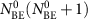, which is negligibly small in this high phonon frequency range. Moreover, these phonon modes in the range where the normalized deviation is non-linear to qx contribute negligibly to actual thermal transport. (See Supplementary Fig. 1)Figure 2: The displaced distribution of phonons at 100 K in reciprocal space of a graphene sheet.

The macroscopic drift motion of phonons can be explained by strong N-scattering compared with R-scattering shown in Fig. 3b. The phonon distribution under a temperature gradient is slightly displaced, meaning that the phonons gain excess momentum from the temperature gradient. Then, the excess momentum is affected by N- and R-scatterings in very different ways24. Through N-scatterings, the excess momentum is exchanged among phonons such that all phonon modes exhibit the same drift velocity and approach the displaced Bose–Einstein distribution in equation (1). However, R-scattering destroys the excess momentum and induces phonon modes to relax to the stationary equilibrium Bose–Einstein distribution. In graphene, N-scattering is much stronger than R-scattering by at least two orders of magnitude in a wide spectrum as shown in Fig. 3b. Therefore, the excess momentum gained from a temperature gradient is redistributed by strong N-scattering without any considerable reduction by R-scattering such that most phonon modes have the same drift velocity regardless of polarization and wavevector.Figure 3: The wide window of sample widths for phonon Poiseuille flow in graphene due to strong N-scattering.

In addition to the macroscopic collective motion of phonons, it is also important to find the specific length and timescales for hydrodynamic transport. If length and timescales approach infinity, the transport is not hydrodynamic unless R-scattering is completely avoided. Owing to the existence of R-scattering, as we previously stated, a temperature gradient is required to maintain the drift motion of phonons in Fig. 2. In an infinitely large graphene sheet under a static temperature gradient, the drift velocity of phonons exhibits a uniform distribution across the sample and its magnitude scales as the magnitude of the temperature gradient, both of which can be explained by Fourier’s law. However, roughly speaking, if the sample size is smaller than the phonon mean free path of R-scattering or the temperature gradient changes with time faster than the R-scattering rate, the transport cannot be described by the diffusive law. In such length and timescales, the transport with macroscopic drift motion shown in Fig. 2 can be hydrodynamic. These considerations regarding length and timescales for hydrodynamic transport lead to the discussion on phonon Poiseuille flow and second sound in the following sections.

The displaced distribution we present here is the first explicit confirmation of the assumption used in past theoretical studies regarding hydrodynamic phonon transport12,13,25,26. It also provides a theoretical basis that we will exploit to examine phonon Poiseuille flow and second sound in the following sections, further highlighting the significant hydrodynamic phonon transport in graphene.

### Phonon Poiseuille flow

Phonon Poiseuille flow refers to the steady-state hydrodynamic phonon transport under a temperature gradient in a sample. The temperature gradient plays a similar role as pressure gradient for the molecular Poiseuille flow. Here it is assumed that the sample is long enough for the heat flux to be fully developed and to be invariant along the flow direction. Owing to their differing sources of thermal resistance, phonon Poiseuille flow is distinctly different from the more well-known diffusive phonon transport. In diffusive phonon transport, thermal resistance is mostly due to R-scattering, which can occur anywhere inside a sample. Therefore, the heat flux in diffusive phonon transport is uniform, as shown in Fig. 1b. On the other hand, the thermal resistance in phonon Poiseuille flow is due to diffuse boundary scattering combined with many N-scatterings, analogous to viscous effects in fluid flow. The drift velocity is small near the boundary because of diffuse boundary scattering, leading to the formation of a drift velocity gradient along the direction perpendicular to heat flow (Fig. 1a). The boundary scattering can be assumed to be diffuse rather than specular even at low temperature such as 100 K. (see Supplementary Fig. 4 and Supplementary Note 2.) The excess momentum of phonons from the temperature gradient is then transferred to the boundary through the drift velocity gradient and many N-scattering events, and finally destroyed by diffuse boundary scattering. Therefore, thermal conductivity largely depends on the rate of momentum transfer to the boundary, a quantity that is determined by N-scattering rates and a sample width, just as resistance in fluid flow depends on viscosity and a pipe diameter. The significance of the extrinsic momentum loss mechanism in the hydrodynamic regime, diffuse boundary scattering, implies that a material’s thermal conductivity largely depends on the sample shape and geometry in contrast to the diffusive case. This is also much different from the ballistic case since the sample size in the hydrodynamic regime is assumed to be much larger than phonon mean free path.

The momentum loss mechanism in phonon Poiseuille flow imposes constraints on sample width in order for phonon Poiseuille flow to occur26. If the width of a sample is too large, the excess momentum is more likely to be destroyed by R-scattering before being transferred to the boundary. In this case, the transport is close to the diffusive regime rather than the hydrodynamic regime. On the other hand, if the sample width is less than the phonon mean free path, transport is ballistic. These considerations determine the upper and the lower bounds, respectively, of the sample width for phonon Poiseuille flow (see Methods and Supplementary Note 3 for details).

A window of sample widths for phonon Poiseuille flow, calculated from first principles, is shown in Fig. 3a. We also present the results for another allotrope of carbon, diamond, for comparison with graphene, since diamond features weak U-scattering compared with N-scattering owing to its large Debye temperature27,28. The figure shows that graphene has a much wider window of sample widths for phonon Poiseuille flow than diamond. The possible range of sample widths in diamond with an extremely enriched isotope content (0.01% 13C) is too narrow even at the lowest temperature we examined (50 K). With a moderately enriched isotope condition (0.1% 13C), there is no possible sample width for which phonon Poiseuille flow would occur. The results for diamond confirm the significant difficulty in observing hydrodynamic phonon transport in three-dimensional materials5. In graphene, however, there is a large window of sample widths at temperatures below 100 K. When the isotope content is increased from 0.01 to 0.1%, the window becomes narrower but is still very wide at temperatures below 100 K.

Graphene’s exceptionally wide range of temperatures and sample widths for phonon Poiseuille flow is due to the strong N-scattering shown in Fig. 3b. We compare the scattering rates of graphene and diamond in (Fig. 3b–e) to highlight the strong N-scattering in graphene. The N-scattering rates in graphene are around 1010 s−1, whereas in diamond they are 108 s−1 at 100 K. Owing to the strong N-scattering in graphene, R-scattering remains comparatively small even when the isotope content matches the naturally occurring case, 1.1% 13C (Fig. 3c). In diamond, however, R-scattering rates are comparable to N-scattering rates at the same isotope content (Fig. 3e). The underlying reasons for the strong N-scattering in graphene will be further discussed later.

For the experimental confirmation of phonon Poiseuille flow, thermal conductivity can be measured by varying sample width. Here we briefly discuss several transport regimes in various sample widths. When the sample width is much larger than the mean free path of R-scattering, transport is diffusive and thermal conductivity does not vary with sample width. As the sample width decreases such that it is smaller than the mean free path of R-scattering but larger than the mean free path of N-scattering, the phonon transport can be described by Poiseuille flow. It is well known that the mass flow rate of molecular Poiseuille flow in two-dimensional duct scales as the third power of duct width. Similarly, in phonon Poiseuille flow, heat flow rate and thermal conductivity scale as the third power and the second power of sample width, respectively. (see Supplementary Note 4 and Supplementary Fig. 7 for details) The thermal conductivity that is superlinearly proportional to sample width makes phonon Poiseuille flow distinguished from the diffusive transport. As the sample width is further decreased and comparable to the mean free path of N-scattering, the transport regime is in between hydrodynamic and ballistic limits. In this case, the phonon system is similar to rarefied gas in a molecule system and it may be possible to observe phonon Knudsen minimum29. The phonon Knudsen minimum was observed in liquid helium where phonons carry most of heat at extremely low temperature30,31. If the sample width is further decreased and much smaller than the phonon mean free path of N-scattering, the transport is ballistic. The thermal conductivity in the ballistic regime described by the Casimir limit cannot be superlinearly proportional to the sample width. The thermal conductivity should be always sublinearly or linearly proportional to the sample width. Therefore, the superlinear dependence of thermal conductivity on sample width can be used for confirming phonon Poiseuille flow.

However, there are several possible challenges in actual experiments. For example, one may need several graphene samples that have different sample widths but have the same quality in terms of isotope content or other defect density. The possible variations in the quality between several samples can make it untrustworthy to deduce any trend of thermal conductivity as a function of sample width. In addition, the superlinear dependence of thermal conductivity on sample width may not be a decisive evidence for hydrodynamic transport. The superlinear dependence was also observed in ballistic transport regime in silicon nanowires with roughened surface32.

Another indication of phonon Poiseuille flow, which has been used in the past work5,33,34, is found in how thermal conductivity changes with temperature. An increase in the thermal conductivity with an exponent in temperature larger than that for the ballistic case is regarded as a direct evidence of phonon Poiseuille flow26,35. In the ballistic regime, thermal conductivity, κ, can be expressed with a simple formula, κ~cvL, where c is a specific heat, v is a group velocity and L is a characteristic size of sample limiting phonon mean free path. Since the sample size, L, is not changed with temperature, an increase of thermal conductivity with temperature should be associated only with specific heat and group velocity, both together determining thermal conductance in the ballistic limit. In the hydrodynamic regime, however, the effective sample size for the boundary scattering increases with temperature. This is because the N-scattering rate increases with temperature, leading to a longer travel distance of phonons to the boundary as discussed in Supplementary Note 3. The effective sample size for boundary scattering as well as the ballistic thermal conductance, cv, increase with temperature. Therefore, the thermal conductivity in the hydrodynamic regime should increase more rapidly than the ballistic thermal conductance increases with temperature. Considering that the thermal conductance of graphene in the ballistic limit increases as T1.68 (ref. 36), an observation of the thermal conductivity increasing with an exponent in temperature much larger than 1.68 can indicate phonon Poiseuille flow.

In recent years, there have been many successful measurements of the thermal conductivity of graphene, but more advances would be required to observe phonon Poiseuille flow. For thermal conductivity measurements using Raman spectroscopy, the temperature range was limited to temperatures above room temperature because of large uncertainties near room temperature37,38,39,40. Alternatively, a microfabricated heater-sensor-assembly was used41,42,43, but the samples were not isotopically enriched and too small to observe phonon Poiseuille flow.

### Second sound

Second sound refers to the propagation of a temperature wave (or phonon density wave) provoked by a heat pulse, analogous to ordinary sound in a fluid, which is pressure wave propagation. The propagation of a heat pulse in the hydrodynamic regime is much different from those in diffusive or ballistic regimes as shown in Fig. 1c,d. The pulse in the hydrodynamic regime is transmitted by many N-scatterings4,9,44,45, whereas the pulse in the diffusive regime is largely damped by R-scattering and cannot propagate. The transmission of a heat pulse can also be observed in ballistic transport, but the sample size is limited to below phonon mean free path. Therefore, the phenomenon of second sound can provide a unique way to transmit a heat pulse without leaving a temperature trace behind the wave front in a sample larger than phonon mean free path. It is important to note that second sound is different from acoustic sound in a solid because the former is a phonon density wave, which is a collective motion of phonons in a wide spectrum maintained by many N-scattering processes, whereas the latter is just ballistic transport of extremely long-wavelength phonons.

The required conditions on temperature and sample size for second sound are determined from the relative strength between N-scattering and momentum-destroying scatterings26. The frequency of second sound or the inverse of a pulse duration time (Ω) should be larger than the combined rate of U-scattering, isotope scattering and boundary scattering (τRB−1, hereafter RB-scattering refers to U-scattering, isotope scattering and boundary scattering combined), but smaller than the N-scattering rate (τN−1).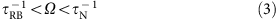The former condition minimizes damping due to RB-scatterings. In particular, boundary scattering is not desirable for second sound, while it provides an important feature of phonon Poiseuille flow. The latter condition in equation (3) allows enough time to form a well-defined phonon density pulse. Otherwise, the phonon excitation would be randomized by N-scattering and would not form a pulse, analogous to the situation where an ordinary sound in a fluid is strongly damped when the sound frequency is high and comparable to the rate of scattering between molecules46.

In Fig. 4a,b, we show a wide range of second sound frequencies in graphene below 100 K. The frequency range of second sound in graphene becomes narrow on the inclusion of isotope and boundary scatterings, but is still considerable when the sample is larger than 100 μm and isotope content is less than 0.1%. However, the frequency range of second sound in diamond does not exist for the given conditions since equation (3) cannot be satisfied. Shown in Fig. 4c,d are contour plots of second sound frequency range for various sample sizes, isotope contents and temperature conditions. In the same examined range of sample sizes, isotope concentration and temperature for Fig. 4c,d, we found that second sound is not possible at all in diamond. Similar to phonon Poiseuille flow, the main difference between graphene and diamond is attributable to the extremely strong N-scattering compared with R-scattering in graphene.Figure 4: The possible frequency ranges of second sound in graphene and diamond.

The second sound has been experimentally confirmed by measuring transient temperature response after applying a heat pulse4,9,44,45,47. One can apply a heat pulse at one side of a sample and measure temperature change with respect to time, dT/dt, at the other side of the sample. If a clear peak in dT/dt is observed, it can be attributed to either ballistic or hydrodynamic transport. The second sound peak can be distinguished from a ballistic pulse using the fact that the propagation of second sound is slower than the propagation of acoustic sound or ballistic phonon transport. For three-dimensional materials, it is theoretically estimated that the speed of second sound, vII, is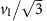where vI is the speed of acoustic sound in Debye model2,48,49. In past experiments, a clear peak in dT/dt was observed with a delay time that could be explained well with the theoretical prediction of the speed of second sound,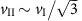.

The second sound in graphene can be measured in a similar way as in the three-dimensional material cases. However, the speed of second sound cannot be estimated asbecause the Debye model is not valid for graphene due to the quadratic ZA branch. Instead of assuming a Debye model, we calculated the speed of second sound using the phonon dispersion determined from first principles. (see Methods for details) The calculated speed of second sound in graphene is plotted in Fig. 5a. In the figure, the speed of second sound ranges from 2,000 to 3,000 m s−1 and slightly increases with temperature. The dependence on temperature is because the group velocity of ZA phonon modes increases with frequency. At low temperatures where the most excited phonons are low-frequency phonons, the average group velocity is small, resulting in slower propagation of second sound than that of the high temperature cases. It would be interesting to see how the speed of second sound is compared with the speed of ballistic transport in graphene. In Fig. 5b, we compare the delay time of ballistic transport pulse and second sound in the heat pulse experiment mentioned above. The delay time of a ballistic transport pulse was calculated based on Landauer’s formalism50. (see Methods for details) In Fig. 5b, there is a significant broadening in the ballistic transport pulse because the group velocity of ZA phonon modes largely depends on frequency. In addition, the ballistic heat pulse becomes faster at higher temperature because high frequency phonons with large group velocity are excited. We also plot the delay time of second sound based on the calculated speed of second sound in Fig. 5a. The delay time of second sound is around three times longer than that of the peak of ballistic heat pulse. The difference in delay time by three times, together with the estimated speed of second sound, can be used to separate second sound signal from ballistic heat pulse.

## Discussion

The large contrast between graphene and diamond regarding hydrodynamic transport indicates that there are more reasons for the significant hydrodynamic transport in graphene, in addition to its large Debye temperature. Here we show that extremely large anharmonicity and density-of-states of long-wavelength ZA phonons, both originating from two-dimensional characteristics, are responsible for the significant hydrodynamic transport.

The strong three-phonon scattering in graphene reflects a large phonon mode anharmonicity. The mode Grüneisen parameters give a measure of this anharmonicity51, and their magnitudes become large as wavelength increases (Fig. 6a). The extremely large magnitude of the Grüneisen parameters of the ZA modes near the zone centre is a characteristic of two-dimensional materials as explained by elasticity theory52. The elasticity theory predicts the divergence of the mode Grüneisen parameter, as γq~−1/|q|2 where γq is a mode Grüneisen parameter at wavevector, q. The actual divergence, however, is prevented by the strong phonon renormalization effect or any in-plane strain that stiffens the ZA phonon dispersion very near the zone centre52,53,54. In general, at large wavelengths, the three-phonon scattering processes are dominated by N-scattering since U-processes by definition require large-wavelength phonons. In particular, due to its large Debye temperature, phonons in graphene are predominantly populated in the large-wavelength region, further making N-scattering much stronger than U-scattering. This strong N-scattering is consistent with the behaviour of the mode Grüneisen parameters for ZA phonons in Fig. 6a and is confirmed in Fig. 3b,c, and also has been previously reported19,55.Figure 6: The two-dimensional features of phonon modes in graphene responsible for the significant hydrodynamic phonon transport.

The large gap between N- and R-scattering rates in graphene shown in Fig. 3b,c is further highlighted when considering the frequency range that mostly contributes to thermal transport. The transport regime, such as hydrodynamic or diffusive, is determined by comparing average scattering rates of N- and R-process as seen in second sound. It is noteworthy that the frequency ranges largely contributing the average scattering rates are different for graphene and diamond, because of their differing phonon density-of-states. The density-of-states is a constant for the quadratic dispersion in two-dimensional materials, while it increases with the square of frequency for the linear dispersion in three-dimensional materials. Therefore, the density-of-states of low-frequency phonons in the former case is much larger than that of the latter case, implying that role of low-frequency phonons in graphene is very significant. In Fig. 6b, we present the cumulative weighting factor for averaging the scattering rates as a function of phonon frequency. (see Methods for the definition of the cumulative weighting factor.) The cumulative weighting factor shows that the frequency ranges that are important for the averaged scattering rate are very different for diamond and graphene. From Fig. 6b, low-frequency phonons are most important for the quadratic dispersion in two-dimensional materials, whereas mid-frequency phonons give a larger contribution for the linear dispersion in three-dimensional materials. The significant role of low-frequency phonons in graphene results in the robust hydrodynamic phonon transport since low-frequency phonons exhibit a larger gap between N- and R-scattering rates as shown in Fig. 3b,c.

In conclusion, we predict the hydrodynamic phonon transport in suspended graphene that is clearly distinguishable from the usual diffusive or ballistic phonon transport. The significant hydrodynamic transport in graphene is attributed to two-dimensional features such as extremely large scattering rates for momentum conserving N-processes and large density-of-states of long-wavelength ZA phonons. The significant hydrodynamic phonon transport in graphene provides a new perspective beyond diffusive and ballistic transport pictures to understand thermal transport in two-dimensional materials. In this work, we have focused on the sub-room temperature range, where hydrodynamic transport dominates over diffusive transport, but the hydrodynamic transport is also important for room temperature cases. The phonon transport in graphene at room temperature cannot be solely described by the diffusion limit due to strong N-scattering55, and the hydrodynamic transport presented here indicates another limit one may need to consider to fully understand phonon transport in graphene at room temperature. In addition, the significant hydrodynamic features imply practical importance. For example, considering the importance of boundary scattering shown in phonon Poiseuille flow, thermal rectification would be achievable in a tapered graphene sheet. The fast thermal transport without damping featured in second sound also shows a potential usage of graphene as thermal interconnects or thermal signal transmitters.

## Methods

### Phonon distribution function from first principles

The second- and third-order force constants of graphene were calculated from the density functional perturbation theory56,57 using the Quantum-Espresso package58. The pseudopotential contains 2s2p2 as valence states with the Perdew–Zunger exchange-correlation functional59. The calculated force constants were validated by comparing mode Grüneisen parameters as in previous studies19,21. (see Supplementary Fig. 2) The force constants of diamond were adopted from ref. 60. The three-phonon scattering rates were calculated using perturbation theory15. The strong renormalization effect for long-wavelength ZA phonons in graphene was included by using phonon stiffening parameters from ref. 52. For the isotope scattering calculation, the 13C isotope was treated as a point defect61. Using the calculated three-phonon and phonon-isotope scattering rates, the linearized Peierls–Boltzmann equation was solved with the iterative method16 for the distribution function under a temperature gradient. For calculating scattering rates and solving the Peierls–Boltzmann equation, the first Brillouin zone was sampled with 70 × 70 and 30 × 30 × 30 meshes for graphene and diamond, respectively.

### The condition for phonon Poiseuille flow

If phonons exhibit the displaced distribution, the transport of all phonon modes can be described by a single parameter, the displacement. Exploiting this feature, the macroscopic momentum balance equation can be derived from the Peierls–Boltzmann equation by taking crystal momentum as a moment26,62. Assuming a two-dimensional material, the momentum balance equation is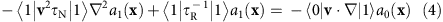where v is a group velocity and isotropic phonon dispersion is assumed. The above equation contains inner products of the eigenstates of the N-scattering operator, |0› and |1›. The eigenstates, |0› and |1›, represent the deviation of phonon distribution due to the temperature variation in real space and to a drift velocity, respectively25. Both eigenstates are expressed as13,25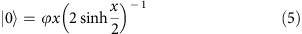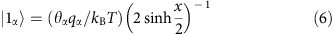where x is the dimensionless phonon frequency, ħω/kBT. The ϕ and θ represent normalization factors for each state and α denotes a direction. The a0(x) and a1(x) in equation (4) are weights of the two states in the actual distribution at position x in real space. The physical meanings of a0(x) and a1(x) are temperature and drift velocity, respectively. The detailed derivation and discussion can be found in refs 13, 25, 26, 62. Equation (4) was originally derived for isotropic and linear dispersion13,26, but then extended to general cases62.

Equation (4) looks similar to the Stokes equation, which is the macroscopic momentum balance equation for molecules when there is no change in the drift velocity along the flow direction, and thus the inertia term in the Navier–Stokes equation is removed. The right-hand side shows momentum gain by the temperature gradient, analogous to the pressure gradient term in the Stokes equation. The first term on the left-hand side means momentum transfer by N-scattering, analogous to the viscous term in the Stokes equation. The second term on the left-hand side reflects the momentum loss by R-scattering and does not have any counterpart in the Stokes equation. Therefore, for phonon Poiseuille flow to occur, the viscous effect, ‹1|v2τN|1›2, should be larger than the R-scattering effect, ‹1|τR−1|1›. To compare the strength of the first and the second terms on the left-hand side, we estimate the 2 operator as (L/2)−2, where L is a sample width. Then, the required condition for the viscous effect being larger than the R-scattering effect is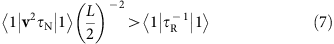Adding the condition for non-ballistic transport to the above gives,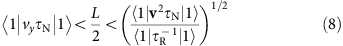where vy is a group velocity along the sample width direction. The above criteria can be qualitatively understood with a random walk picture of phonon transport26,35. (see Supplementary Note 3) For phonon Poiseuille flow, second sound and spectral contribution to an averaged scattering rate calculations, we consider only the lowest phonon branches for graphene (ZA) and diamond (TA1), respectively, since these branches carry most of heat at low temperature55,63, also as confirmed in our calculation. (see Supplementary Fig. 1)

### The condition for second sound

The N- and RB-scattering rates calculated from first principles were averaged with the displaced state, |1› (refs 26, 62).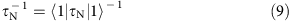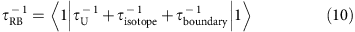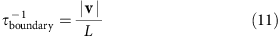In the above expressions, we made a small modification from the original expression given by ref. 26. Please see Supplementary Note 5 for details.

### Derivation of the speed of second sound

The past studies theoretically estimated the speed of second sound in three-dimensional materials aswhere vI is the speed of acoustic sound in Debye model2,48,49. For graphene, however, its phonon dispersion cannot be described by Debye model because of the nearly quadratic ZA phonon branch. Here we derive the wave equation for second sound from the Peierls–Boltzmann equation for arbitrary phonon dispersion cases to estimate the speed of second sound. We assume that R-scattering is negligibly weak and thus crystal momentum is approximately conserved.

We start from the Peierls–Boltzmann transport equation for phonon transport.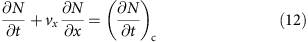where the right-hand side represents the change in the phonon distribution due to scattering. Taking energy and crystal momentum along the flow direction as a moment leads to the macroscopic equations for energy and momentum balance, respectively.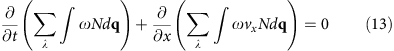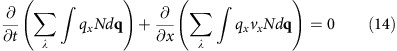where λ is the phonon polarization. The right-hand side of equation (13) is zero because energy is always conserved on scatterings. The right-hand side of equation (14) is also zero because here we assume R-scattering is negligibly weak and thus crystal momentum is conserved. The actual phonon distribution (N) under very weak R-scattering can be approximated as the displaced distribution function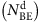as we confirmed in Fig. 2. The displaced distributioncan be linearized because the displacement in phonon distribution has a very small value: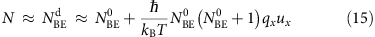In addition, we can neglect higher-order terms associated with the small displacement (ux) in equations (13) and (14). The resulting equations are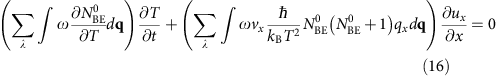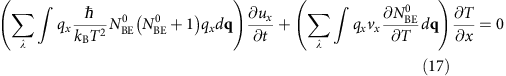Taking the time derivative of equation (16) and the spatial derivative of equation (17), and then subtracting these two equations result in the hyperbolic wave equation for second sound.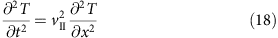where vII represents the speed of second sound and can be expressed as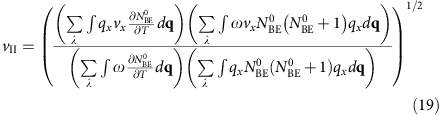The above expression can be simplified using the notation, |0› and |1›.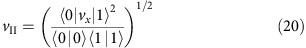Applying the Debye model for three-dimensional materials into equation (20) gives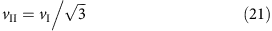as previously derived2,48,49. For graphene, we calculate the speed of second sound using equation (20) and the phonon dispersion of ZA branch from first principles. We also calculated the speed of second sound by including all three acoustic branches for the inner products in equation (20), but the speed of second sound is almost same as that by including only ZA branch.

### Delay time of ballistic heat pulse

Unlike other three-dimensional materials, the speed of ballistic transport in graphene cannot be represented by a single value of the speed of acoustic sound because of the quadratic ZA phonon branch. The group velocity, which largely depends on phonon frequency, can cause a significant broadening in a ballistic heat pulse. To take account of this effect, we suppose an experiment for observing second sound, similar to the past experiments for three-dimensional materials4,9,45,47. Suppose we have a rectangular shape graphene sheet with a point heat source at the left edge and a point thermal sensor at the right edge, as illustrated in the inset of Fig. 5b. At t=0, a heat pulse is generated at the point source with a delta function profile with respect to time. Assuming that all phonon modes transport ballistically, then the temperature change at the sensor can be expressed using the Landauer’s formalism50.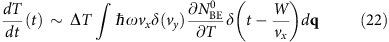where W is a distance between the source and the sensor. The ΔT is a temperature difference between the source and the sensor at t=0+. The delta function of vy is included because phonon modes propagating with an oblique angle to the x direction cannot contribute to the ballistic thermal transport between the point source and the point sensor. This constraint prevents any additional broadening of the ballistic heat pulse from the finite size of the source and the sensor. In the actual cases where the source and the sensor have finite size, the temperature signal should be broader than that given by equation (22). Using equation (22) and phonon dispersion of ZA modes from first principles, we plot the temperature signal in ballistic transport in Fig. 5b.

### Spectral contribution to the averaged scattering rate

In the macroscopic transport equation like equation (4), the scattering rate is averaged using the displaced state,|1›.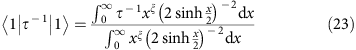Similarly, we define the cumulative weighting factor for averaging scattering rate as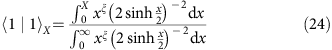where ξ is one for quadratic dispersion in two-dimensional materials and four for linear dispersion in three-dimensional materials. The values of ξ can be derived from the definition of the state, |1›, in equations (5) and (6) by assuming isotropic phonon dispersion. In fact, for the case of perfectly quadratic dispersion in two-dimensional materials (ξ=1), the low-frequency contribution to the averaged scattering rate diverges as frequency approaches zero. Such a singular behaviour is prevented by phonon stiffening from the renormalization effect. The spectral contribution in Fig. 6b includes the renormalization effect by using phonon stiffening parameters given in ref. 52.

How to cite this article: Lee, S. et al. Hydrodynamic phonon transport in suspended graphene. Nat. Commun. 6:6290 doi: 10.1038/ncomms7290 (2015).

## References

1. 1

Casimir, H. B. G. Note on the conduction of heat in crystals. Physica 5, 495–500 (1938).

2. 2

Ward, J. C. & Wilks, J. III. Second sound and the thermo-mechanical effect at very low temperatures. Philos. Mag. 43, 48–50 (1952).

3. 3

De Haas, W. J. & Biermasz, T. The thermal conductivity of KBr, KCl and SiO2 at low temperatures. Physica 4, 752–756 (1937).

4. 4

Ackerman, C. C., Bertman, B., Fairbank, H. A. & Guyer, R. A. Second sound in solid helium. Phys. Rev. Lett. 16, 789–791 (1966).

5. 5

Mezhov-Deglin, L. Measurement of the thermal conductivity of crystalline He4. J. Exp. Theor. Phys. 49, 66–79 (1965).

6. 6

Dresselhaus, M. et al. New directions for low-dimensional thermoelectric materials. Adv. Mater. 19, 1043–1053 (2007).

7. 7

Cahill, D. G. et al. Nanoscale thermal transport. J. Appl. Phys. 93, 793–818 (2003).

8. 8

Cahill, D. G. et al. Nanoscale thermal transport. II. 2003–2012. Appl. Phys. Rev. 1, 011305 (2014).

9. 9

Jackson, H. E., Walker, C. T. & McNelly, T. F. Second sound in NaF. Phys. Rev. Lett. 25, 26–28 (1970).

10. 10

Vincenti, W. G. & Kruger, C. H. Introduction to Physical Gas Dynamics Wiley (1965).

11. 11

Klemens, P. Thermal conductivity of solids at low temperatures. Handbuch der Physik 14, 198–281 (1956).

12. 12

Sussmann, J. A. & Thellung, A. Thermal conductivity of perfect dielectric crystals in the absence of Umklapp processes. Proc. Phys. Soc. 81, 1122 (1963).

13. 13

Guyer, R. A. & Krumhansl, J. A. Solution of the linearized phonon Boltzmann equation. Phys. Rev. 148, 766–778 (1966).

14. 14

Deinzer, G., Birner, G. & Strauch, D. Ab initio calculation of the linewidth of various phonon modes in germanium and silicon. Phys. Rev. B 67, 144304 (2003).

15. 15

Broido, D. A., Malorny, M., Birner, G., Mingo, N. & Stewart, D. A. Intrinsic lattice thermal conductivity of semiconductors from first principles. Appl. Phys. Lett. 91, 231922 (2007).

16. 16

Omini, M. & Sparavigna, A. Beyond the isotropic-model approximation in the theory of thermal conductivity. Phys. Rev. B 53, 9064–9073 (1996).

17. 17

Fugallo, G., Lazzeri, M., Paulatto, L. & Mauri, F. Ab initio variational approach for evaluating lattice thermal conductivity. Phys. Rev. B 88, 045430 (2013).

18. 18

Esfarjani, K., Chen, G. & Stokes, H. T. Heat transport in silicon from first-principles calculations. Phys. Rev. B 84, 085204 (2011).

19. 19

Bonini, N., Garg, J. & Marzari, N. Acoustic phonon lifetimes and thermal transport in free-standing and strained graphene. Nano Lett. 12, 2673–2678 (2012).

20. 20

Lindsay, L., Broido, D. A. & Reinecke, T. L. First-principles determination of ultrahigh thermal conductivity of boron arsenide: a competitor for diamond? Phys. Rev. Lett. 111, 025901 (2013).

21. 21

Lee, S., Esfarjani, K., Mendoza, J., Dresselhaus, M. S. & Chen, G. Lattice thermal conductivity of Bi, Sb, and Bi-Sb alloy from first principles. Phys. Rev. B 89, 085206 (2014).

22. 22

Lee, S., Esfarjani, K., Luo, T., Zhou, J., Tian, Z. & Chen, G. Resonant bonding leads to low lattice thermal conductivity. Nat. Commun. 5, 3525 (2014).

23. 23

Lindsay, L., Li, W., Carrete, J., Mingo, N., Broido, D. A. & Reinecke, T. L. Phonon thermal transport in strained and unstrained graphene from first principles. Phys. Rev. B 89, 155426 (2014).

24. 24

Callaway, J. Model for lattice thermal conductivity at low temperatures. Phys. Rev. 113, 1046 (1959).

25. 25

Krumhansl, J. A. Thermal conductivity of insulating crystals in the presence of normal processes. Proc. Phys. Soc. 85, 921 (1965).

26. 26

Guyer, R. A. & Krumhansl, J. A. Thermal conductivity, second sound, and phonon hydrodynamic phenomena in nonmetallic crystals. Phys. Rev. 148, 778–788 (1966).

27. 27

Wei, L., Kuo, P. K., Thomas, R. L., Anthony, T. R. & Banholzer, W. F. Thermal conductivity of isotopically modified single crystal diamond. Phys. Rev. Lett. 70, 3764–3767 (1993).

28. 28

Ward, A., Broido, D. A., Stewart, D. A. & Deinzer, G. Ab initio theory of the lattice thermal conductivity in diamond. Phys. Rev. B 80, 125203 (2009).

29. 29

Chen, G. Nanoscale Energy Transport and Conversion: A Parallel Treatment of Electrons, Molecules, Phonons, and Photons Oxford Univ. Press (2005).

30. 30

Greywall, D. S. Thermal-conductivity measurements in liquid 4He below 0.7 K. Phys. Rev. B 23, 2152–2168 (1981).

31. 31

Whitworth, R. W. Experiments on the flow of heat in liquid helium below 0.7 K. Proc. R. Soc. Lond. A 246, 390–405 (1958).

32. 32

Hochbaum, A. I. et al. Enhanced thermoelectric performance of rough silicon nanowires. Nature 451, 163–167 (2008).

33. 33

Thomlinson, W. C. Evidence for anomalous phonon excitations in solid He3. Phys. Rev. Lett. 23, 1330–1332 (1969).

34. 34

Hogan, E. M., Guyer, R. A. & Fairbank, H. A. Thermal conductivity of oriented single crystals of hexagonal close-packed helium 4. Phys. Rev. 185, 356–373 (1969).

35. 35

Gurzhi, R. Thermal conductivity of dielectrics and ferrodielectrics at low temperatures. J. Exp. Theor. Phys. 46, 719–724 (1964).

36. 36

Bae, M.-H. et al. Ballistic to diffusive crossover of heat flow in graphene ribbons. Nat. Commun. 4, 1734 (2013).

37. 37

Balandin, A. A. et al. Superior thermal conductivity of single-layer graphene. Nano Lett. 8, 902–907 (2008).

38. 38

Ghosh, S. et al. Extremely high thermal conductivity of graphene: prospects for thermal management applications in nanoelectronic circuits. Appl. Phys. Lett. 92, 151911 (2008).

39. 39

Chen, S. et al. Thermal conductivity of isotopically modified graphene. Nat. Mater. 11, 203–207 (2012).

40. 40

Cai, W. et al. Thermal transport in suspended and supported monolayer graphene grown by chemical vapor deposition. Nano Lett. 10, 1645–1651 (2010).

41. 41

Pettes, M. T., Jo, I., Yao, Z. & Shi, L. Influence of polymeric residue on the thermal conductivity of suspended bilayer graphene. Nano Lett. 11, 1195–1200 (2011).

42. 42

Wang, Z. et al. Thermal transport in suspended and supported few-layer graphene. Nano Lett. 11, 113–118 (2010).

43. 43

Xu, X. et al. Length-dependent thermal conductivity in suspended single-layer graphene. Nat. Commun. 5, 3689 (2014).

44. 44

Ackerman, C. C. & Overton, W. C. Second sound in solid helium-3. Phys. Rev. Lett. 22, 764–766 (1969).

45. 45

McNelly, T. F. et al. Heat pulses in NaF: onset of second sound. Phys. Rev. Lett. 24, 100–102 (1970).

46. 46

Kinsler, L. E., Frey, A. R., Coppens, A. B. & Sanders, J. V. Fundamentals of Acoustics 4th edn Wiley-VCH (1999).

47. 47

Narayanamurti, V. & Dynes, R. C. Observation of second sound in bismuth. Phys. Rev. Lett. 28, 1461–1465 (1972).

48. 48

Landau, L. The theory of superfluidity of helium II. J. Phys. USSR 5, 71–90 (1941).

49. 49

Ward, J. & Wilks, J. The velocity of second sound in liquid helium near the absolute zero. Philos. Mag. 42, 314–316 (1951).

50. 50

Imry, Y. & Landauer, R. Conductance viewed as transmission. Rev. Mod. Phys. 71, S306–S312 (1999).

51. 51

Leibfried, G. & Schlömann, G. Heat conduction in electrically insulating crystals. Nachr. Ges. Wiss. Goett. Math.-Phys. K1. 2, 71 (1954).

52. 52

Roldán, R., Fasolino, A., Zakharchenko, K. V. & Katsnelson, M. I. Suppression of anharmonicities in crystalline membranes by external strain. Phys. Rev. B 83, 174104 (2011).

53. 53

de Andres, P. L., Guinea, F. & Katsnelson, M. I. Bending modes, anharmonic effects, and thermal expansion coefficient in single-layer and multilayer graphene. Phys. Rev. B 86, 144103 (2012).

54. 54

Mariani, E. & von Oppen, F. Flexural phonons in free-standing graphene. Phys. Rev. Lett. 100, 076801 (2008).

55. 55

Lindsay, L., Broido, D. A. & Mingo, N. Flexural phonons and thermal transport in graphene. Phys. Rev. B 82, 115427 (2010).

56. 56

Gonze, X. First-principles responses of solids to atomic displacements and homogeneous electric fields: Implementation of a conjugate-gradient algorithm. Phys. Rev. B 55, 10337–10354 (1997).

57. 57

Gonze, X. & Lee, C. Dynamical matrices, Born effective charges, dielectric permittivity tensors, and interatomic force constants from density-functional perturbation theory. Phys. Rev. B 55, 10355–10368 (1997).

58. 58

Giannozzi, P. et al. QUANTUM ESPRESSO: a modular and open-source software project for quantum simulations of materials. J. Phys.: Condens. Matter 21, 395502 (2009).

59. 59

Perdew, J. P. & Zunger, A. Self-interaction correction to density-functional approximations for many-electron systems. Phys. Rev. B 23, 5048–5079 (1981).

60. 60

Broido, D. A., Lindsay, L. & Ward, A. Thermal conductivity of diamond under extreme pressure: a first-principles study. Phys. Rev. B 86, 115203 (2012).

61. 61

Tamura, S.-i. Isotope scattering of dispersive phonons in Ge. Phys. Rev. B 27, 858–866 (1983).

62. 62

Gurevich, V. L. v. Transport in Phonon Systems Elsevier Science Publishers (1986).

63. 63

Seol, J. H. et al. Two-dimensional phonon transport in supported graphene. Science 328, 213–216 (2010).

## Acknowledgements

We thank N. Hadjiconstantinou, L. Shi, B. Liao, M. Luckyanova, C. Landon, D.H. Yi and A. Maznev for useful discussions. We also thank L. Lindsay for providing us force constants of diamond. This work was partially supported by ‘Solid State Solar-Thermal Energy Conversion Center (S3TEC),’ an Energy Frontier Research Center funded by the U.S. Department of Energy, Office of Science, Office of Basic Energy Sciences under Award Number: DE-SC0001299/DE-FG02-09ER46577 (for developing first-principles phonon transport simulation tools) and partially supported by U.S. Department of Defense AFOSR MURI via Ohio State University (for exploring cryogenic applications of phonon transport). S.L. acknowledges support from a Samsung scholarship.

## Author information

Authors

### Contributions

S.L., D.B. and K.E. performed the first-principles calculations and S.L., D.B. and G.C. analysed the data. S.L., D.B. and G.C. wrote the manuscript and G.C. supervised the research. All authors commented on, discussed and edited the manuscript.

### Corresponding author

Correspondence to Gang Chen.

## Ethics declarations

### Competing interests

The authors declare no competing financial interests.

## Supplementary information

### Supplementary Information

Supplementary Figures 1-7, Supplementary Notes 1-5 and Supplementary References (PDF 769 kb)

## Rights and permissions

Reprints and Permissions

• ### Phonon-engineered extreme thermal conductivity materials

• Xin Qian
• , Jiawei Zhou
•  & Gang Chen

Nature Materials (2021)

• ### Non-Fourier phonon heat conduction at the microscale and nanoscale

• Gang Chen

Nature Reviews Physics (2021)

• ### Nonlocal heat conduction in silicon nanowires and carbon nanotubes

• Mingtian Xu

Heat and Mass Transfer (2021)

• ### Nonlocal and nonlinear effects in hyperbolic heat transfer in a two-temperature model

• A. Sellitto
• , I. Carlomagno
•  & M. Di Domenico

Zeitschrift für angewandte Mathematik und Physik (2021)

• ### Heat vortex in hydrodynamic phonon transport of two-dimensional materials

• Man-Yu Shang
• , Chuang Zhang
• , Zhaoli Guo
•  & Jing-Tao Lü

Scientific Reports (2020)